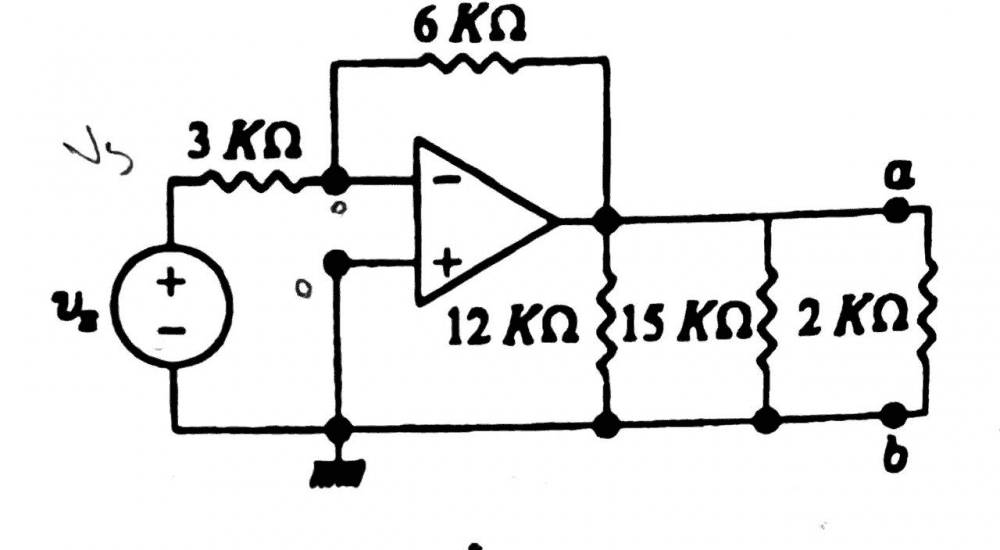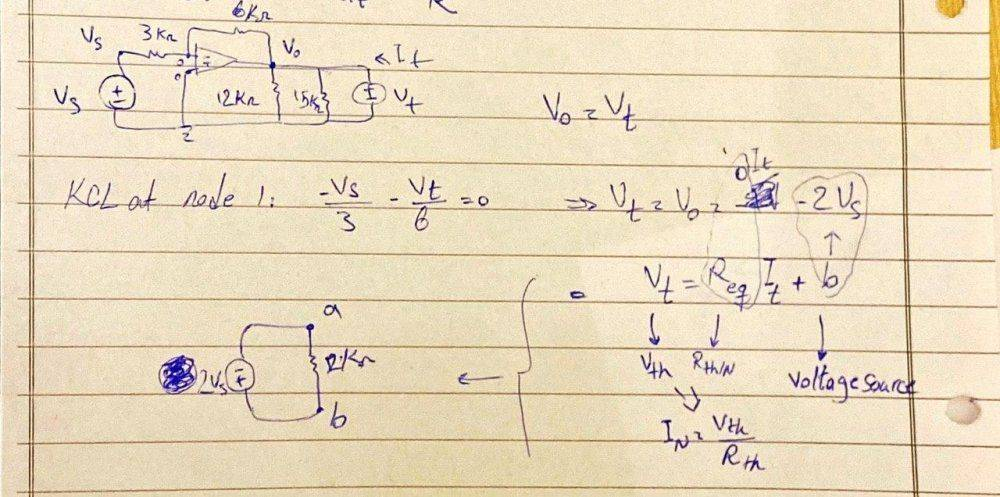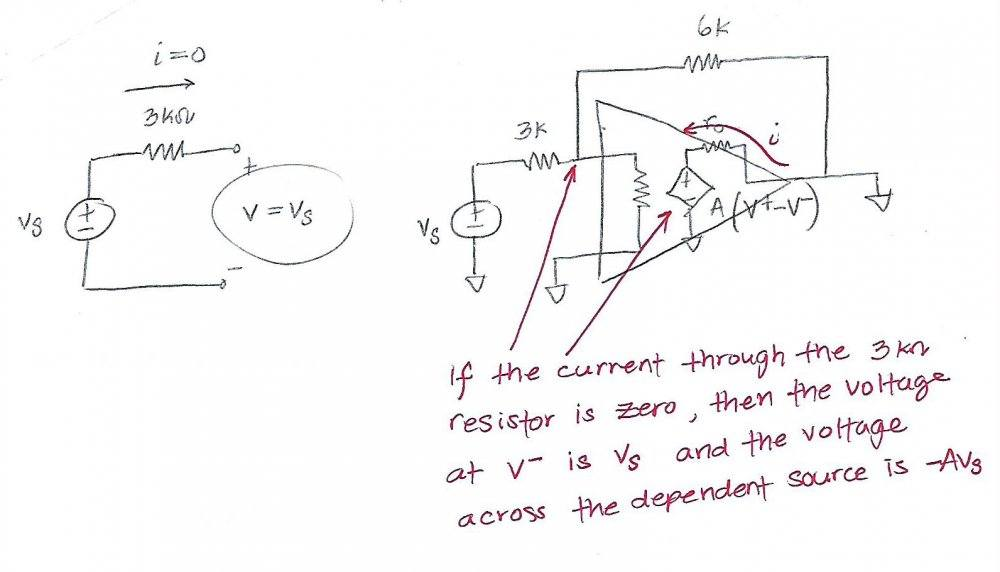# KCL got declined in Norton circuit of an ideal amplifier circuit

Summary:
KCL got declined while trying to find Inorton of an op amp circuit
Hi.
Let's say we have a circuit like that, and we want to find the Thevenin/Norton circuit from a and b points.

So for Vth, we calculate the Voc, in which we remove the 2K ohm resistor and calculate the Voc. I calculated it correctly. But I have problem in calculating the Norton current. So in the Norton circuit, we should calculate Isc, where we remove the 2K ohm resistor and put a wire instead of it.

So in the Norton circuit, the wire would connect ground to Voutput of the amplifier, so the voltage of Voutput would be zero. So as long as we have no potential difference at the two ends of the resistors, no current would pass the 12K and 15K ohm resistors. Also the voltage of the right end of the 6K ohm resistor is also zero (because Voutput = 0v/gnd).

The positive pin of the amplifier is connected to ground and as long as it's an ideal amplifier, the voltage of the negative pin is also considered as 0v due to the positive pin, which means the both ends of the 6K ohm resistor is 0v and no current should pass the 6K ohm resistor.

Now let's write KCL at the negative pin node. The voltage of this node is 0 due to the positive pin, also no current enters the amplifier as it's an ideal one. We have one current from the 3K ohm resistor (one end Vs and the other end gnd, so we have a current entering the node). We have 3 branches at this node, one has current entering the node, one goes to the amplifier and doesn't have current as it's an ideal op amp and one leads to the 6K ohm resistor, but the voltage of both ends of the 6K ohm resistor is zero and the current doesn't flow in that breach either.

So KCL says: -Vs/3K = 0, which declines the law, because we have an actual known voltage from the voltage source and a number never would be equal to zero.
Why KCL got declined here? What's wrong with my solution?Last edited by a moderator:

phyzguy
Thevenin's theorem and Norton's theorem don't work in active circuits which have gain. They only apply to circuits with linear elements such as resistors, voltage sources, and current sources.

•Delta2 and berkeman
cnh1995
Homework Helper
Gold Member
Summary:: KCL got declined while trying to find Inorton of an op amp circuit

Why KCL got declined here
I believe that is because you tried forcing the op-amp output to be zero. The output voltage of the op-amp is fixed at -2Vs. This created a contradiction. You can replace the op-amp output voltage with a constant voltage source.
By shorting the 2k resistor, you are shorting an ideal voltage source, which is an invalid circuit condition.

Thevenin/Norton would not work in this circuit between points a and b.

Thevenin's theorem and Norton's theorem don't work in active circuits which have gain. They only apply to circuits with linear elements such as resistors, voltage sources, and current sources.
Still considered linear. Op amp can be replaced with a VCVS element (Dependent Voltage Source).

phyzguy
OK, but then you can't assume that the two input voltages are equal.

Thevenin/Norton would not work in this circuit between points a and b.
It would. I actually realized the issue.

So basically, in order to create the Thevenin/Norton circuit, you have two find at least two of these:
Req/Vth/In.

As you stated, Vth is -2Vs obviously. We had problem finding In. But for Req, we turn off the independent sources in the circuit, so once we turn off the voltage source, the 3K resistor would get shorted and as it's parallel to other resistors, the Req would be 0 (because the resistance of the 3K ohm resistor would become 0 once it gets shorted). So the thevenin circuit would be an independent source only (V = -2Vs).

There is also another way to find the Thevenin voltage and/or Norton current, where you replace the load network with a test source (Vtest and Itest), and once you solve it, the answer would be something like: Vtest = RItest + b. Vtest = Vth, R = Req, In = Vth/Req, and b is the Voltage Source.So if we use this method and find the answer, R would be 0 and b would be -2Vs, indicating a voltage source-only circuit. Which means we can still find a th/n circuit for it.

Therefore, it's reasonable that KCL/KVL got declined in the Norton circuit, because the resistance tends to 0 and In tends to infinity.

Disregarding this problem, is it valid to say if KVL/KCL gets declined in a linear circuit, it doesn't mean we don't have a Thevenin/Norton circuit, instead it means at least one of the elements, tends to 0/infinty?

Baluncore
Let's say we have a circuit like that, and we want to find the Thevenin/Norton circuit from a and b points.
So in the Norton circuit, we should calculate Isc, where we remove the 2K ohm resistor and put a wire instead of it.
Is it surprising that it falls over when you short circuit the point you are trying to analyse?

You are throwing equations at the circuit in the hope it will solve a problem.
But you have not specified what you are trying to evaluate, or why.

Is the 2k resistor with nodes b=zero and a, an external load or part of the amplifier circuit?

So KCL says: -Vs/3K = 0, which declines the law, because we have an actual known voltage from the voltage source and a number never would be equal to zero. Why KCL got declined here? What's wrong with my solution?

I can't see the relationship between this problem and Thevenin/Norton's theorem.

Let's see an example as follows : -

There is an ideal voltage source V connecting to a potential divider R1 and R2, the voltage across R2 should be equal to V*R2/(R1+R2), now I try to short-circuit the ideal voltage source by putting a perfectly conducting wire across it, so the output voltage of the ideal voltage source is forced to zero, then the voltage across the R2 becomes zero too, but this violates the KVL, because V is an ideal voltage source so the voltage across R2 must be equal V*R2/(R1 R2) according to KVL.

The argument of this example may be very absurd, but seems it can simulate the situation here to some extent.

Since the output of an ideal operational amplifier is equivalent to an ideal voltage source, it cannot be forced to zero by short-circuiting with an perfectly conducting wire, otherwise it will cause conflicts and errors in circuit analysis.

Last edited:
Joshy
Gold Member
Great question! I was definitely baffled at something like this while exploring one of my favourite theorems, and I was even asked a question like this during a job interview.

Your first way works too. Inside of that op-amp it's modeled like a voltage controlled current source. In the analysis you saw that if the current going across the ##3~k\Omega## resistor is zero, then you come across a problem, but it does not mean that ##v_s = 0## and KCL is "broken". The problem is that the positive terminal wants to have the same voltage as the negative terminal, which was one of your assumptions about the op-amp. If the voltage across the two terminals are not zero then the voltage controlled current source inside of it starts sucking in current trying pull the voltage to be the same, and so ##i_{sc}## goes to infinity.

If the voltage across the two terminals are not zero then the voltage controlled current source inside of it starts sucking in current trying pull the voltage to be the same, and so iscisci_{sc} goes to infinity.
Would you please explain this part?

Joshy
Gold Member
I may have made a mistake when I said voltage controlled current source. After reviewing my textbooks it appears it should have been a voltage controlled voltage source and I am not sure if a current source would be acceptable or not for the model; however: neither negate my earlier statements.Inside of the op-amp is a dependent source. That source is dependent on the voltage across the input terminals of the op-amp and it has really high gain ##A##. Note I drew a resistor in between the input terminal it's usually either a very high value sometimes simply an open circuit. When there's zero current going through the 3k resistor it's not that KCL has broken it's that the premise of the two input terminals is no longer true and there's a voltage drop. The reason that the analysis assumes that the voltage is the same is not because there's a mysterious short inside of the op-amp it's because the dependent source tries to pull the voltages to be the same in the case where it's ground it's trying to null it rather than seeing it as a short.

edit:

Didn't want to necro the old post, but I just tried this out with a voltage controlled current source and it's still completely acceptable. I just tried it with a less complicated circuit to confirm the model works.

Last edited:
Still considered linear. Op amp can be replaced with a VCVS element (Dependent Voltage Source).

Even if you do that you can’t solve for Isc like KCL requires because node a would be driven by an ideal vcvs and it can source infinite current. In the original circuit, if you give the opamp a finite output resistance then you can solve for the KCL equivalent circuit.

Disregarding this problem, is it valid to say if KVL/KCL gets declined in a linear circuit, it doesn't mean we don't have a Thevenin/Norton circuit, instead it means at least one of the elements, tends to 0/infinty?

Your math and technique is correct but you solved for the properties of an ideal opamp model, which has zero output impedance and can source infinite current, which matches your answer. If you want a more realistic answer you need a more realistic model of the opamp. In particular it needs a finite output impedance.